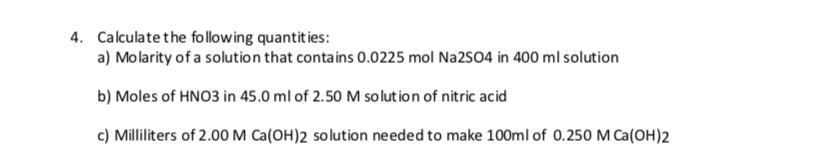# Calculate the follow ing quantit ies:4.a) Molarity of a solution that contains 0.0225 mol Na2SO4 in 400 ml solutionb) Moles of HNO3 in 45.0 ml of 2.50 M solution of nitric acidc) Milliliters of 2.00 M Ca(OH)2 solution ne eded to make 100ml of 0.250 M Ca(OH)2

Question
10 viewshelp_outlineImage TranscriptioncloseCalculate the follow ing quantit ies: 4. a) Molarity of a solution that contains 0.0225 mol Na2SO4 in 400 ml solution b) Moles of HNO3 in 45.0 ml of 2.50 M solution of nitric acid c) Milliliters of 2.00 M Ca(OH)2 solution ne eded to make 100ml of 0.250 M Ca(OH)2 fullscreen
check_circle

Step 1

Equation for calculating mol...

### Want to see the full answer?

See Solution

#### Want to see this answer and more?

Solutions are written by subject experts who are available 24/7. Questions are typically answered within 1 hour.*

See Solution
*Response times may vary by subject and question.
Tagged in

### General Chemistry